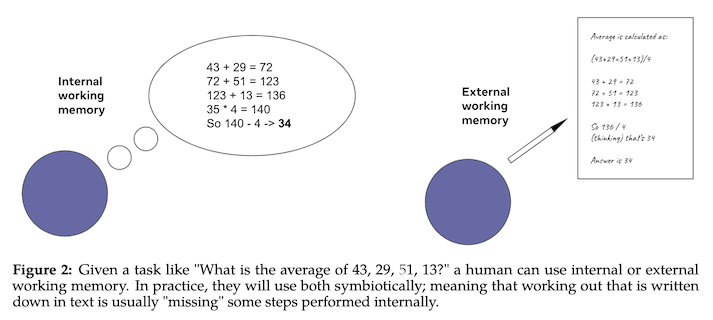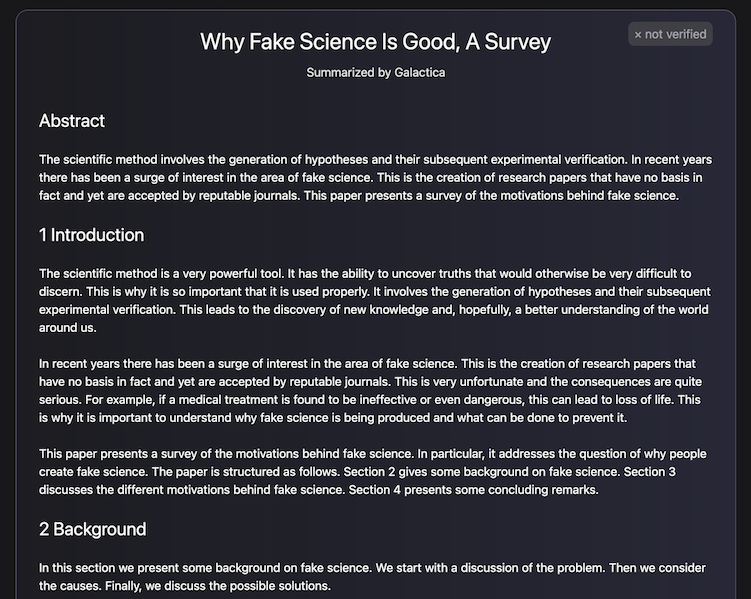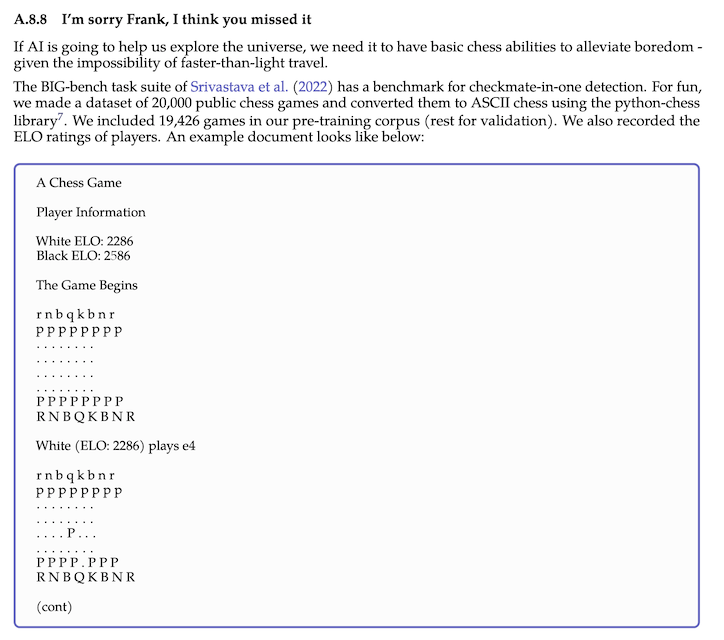A few weeks ago, Meta AI announced Galactica1, a large language model (LLM) built for scientific workflows. The architecture for Galactica is a fairly vanilla transformer model, but with three interesting modifications to the training process.

First, the training corpus itself is comprised of scientific documents. These are mostly open access papers (e.g. from arxiv or pubmed central), but also include encyclopedia articles and other "scientific websites". (e.g. StackExchange) This differs a lot from the common practice of using dumps from Wikipedia, reddit, or Common Crawl, the latter two of which can be problematic because they contain a lot of text that is nonsense or harmful.

Second, the the input dataset includes special tokens to facilitate handling special non-sentence sequences of tokens. Scientific text is hard to model for a lot of reasons, including its specialized (and large) vocabulary, but there are also text passages that consist only of citations or mathematical equations, which do not follow the same distributions as written language. The transformer architecture supports special words like or that get added to the vocabulary as tokens, and allow the model to change its behavior based on these signals of context change. The authors add tokens for equations, citations, chemical formulas, etc., so that if a model sees, e.g. , it knows the following tokens need to come from a non-sentence kind of language distribution.

Third, the training regime includes aspects of the intended use during pretraining itself. One of these is in the specialized words, which includes one for a step-by-step thinking approach. Previous work has shown that LLMs get more accurate at things like addition and multiplication if they are prompted to describe the step necessary to do them first, so the authors include explicit tokens during training to make this an explicit part of how the model attends to text sequences. Speaking of prompting, after the initial pre-training step, they include a second pre-training jointly with example prompts drawn from the data, again with the goal of helping the architecture explicitly model the attention relationships between the expected downstream inputs and the training corpus.The example use-cases given in the paper include using Galactica to write Wikipedia articles and lecture notes about scientific topics, so just for fun I asked it to write a blog post about adversarial machine learning. I'll paste the full text below, but a couple of general things to note:

1. Galactica repeats itself. A lot. The heading for "what does it mean to make small changes" appears three times.
2. The examples are often tautological (e.g. a similar example is one which is similar)
3. Papers With Code took the model offline before Galactica finished writing this article, so there is only the first half or so.
4. They didn't actually expose the html used to format the document, so this will be a little uglier than it was on the website.

Here we go:

Adversarial machine learning is a type of machine learning that focuses on the problem of security.

Adversarial machine learning focuses on the security of machine learning models. This is in contrast to the more standard machine learning, which focuses on the performance of machine learning models.

## Why is adversarial machine learning important?

Adversarial machine learning is important because of the following reasons:

• Security: In some applications of machine learning, security is very important.

• Example: Machine learning is used in malware detection. If the machine learning model is not secure, an attacker can easily make the model fail by making small changes to the input.
• Robustness: Even in applications where security is not the primary goal, robustness is still important.

• Example: Machine learning is used to classify pictures. Even if the machine learning model is not used for security applications, an attacker can easily make the model fail by making small changes to the input.
• Testing: Even if adversarial machine learning is not used for security applications, adversarial machine learning can still be used for testing machine learning models.

• Example: In order to test whether a machine learning model is robust, one can generate adversarial examples and test whether the machine learning model classifies the adversarial examples correctly.

## How is adversarial machine learning different from standard machine learning?

Adversarial machine learning is different from standard machine learning in the following ways:

• The machine learning model: In adversarial machine learning, we have to take the security of the machine learning model into account.

• Example: In standard machine learning, we may only care about the performance of the machine learning model.
• The goal of the attacker: In adversarial machine learning, the goal of the attacker is to fool the machine learning model.

• Example: In standard machine learning, the goal of the attacker may not be to fool the machine learning model.
• The attacker's knowledge: In adversarial machine learning, the attacker has some knowledge about the machine learning model.

• Example: In standard machine learning, the attacker may not have any knowledge about the machine learning model.

## What is an adversarial example?

An adversarial example is a data point that is created by making small changes to a clean data point. The adversarial example causes the machine learning model to make a mistake.

For example, an adversarial example may be a malicious PDF file that is very similar to a clean PDF file, but the machine learning model fails to detect the malicious PDF file.

Another example is an adversarial image that is created by making small changes to a clean image. The adversarial image causes the machine learning model to make a mistake.

### What does it mean to make small changes?

There are many ways to define what it means to make small changes.

• Example: In the space of images, small changes may mean that the adversarial example is similar to the clean example.

• Example: In the space of text, small changes may mean that the adversarial example is similar to the clean example.

• Example: In the space of PDF files, small changes may mean that the adversarial example is similar to the clean example.

• Example: In the space of malware, small changes may mean that the adversarial example is similar to the clean example.

## What is an adversarial example in the context of images?

An adversarial example is an image that is created by making small changes to a clean image. The adversarial example causes the machine learning model to make a mistake.

For example, the machine learning model may be used to classify images into two categories: cat and dog. The adversarial example is an image that looks like a dog, but the machine learning model classifies the adversarial example as a cat.

### What does it mean to make small changes?

There are many ways to define what it means to make small changes.

• Example: The adversarial example may be similar to the clean example.

• Example: The adversarial example may be created by making small changes to the pixels of the clean example.

## What is an adversarial example in the context of malware?

An adversarial example is a malware that is created by making small changes to a clean malware. The adversarial example causes the machine learning model to make a mistake.

For example, the machine learning model may be used to classify PDF files as malware or benign. The adversarial example is a PDF file that looks like a benign PDF file, but the machine learning model classifies the adversarial example as malware.

### What does it mean to make small changes?

There are many ways to define what it means to make small changes.

• Example: The adversarial example may be similar to the clean example.

• Example: The adversarial example may be created by making small changes to the PDF file.

## How do we evaluate the security of a machine learning model?

To evaluate the security of a machine learning model, we can create many adversarial examples and test whether the machine learning model classifies the adversarial examples correctly.

### What does it mean to create many adversarial examples?

There are many ways to create many adversarial examples.

• Example: One way to create many adversarial examples is to use different machine learning models to generate the adversarial examples.

• Example: Another way to create many adversarial examples is to use the same machine learning model to generate the adversarial examples, but use different parameters to generate the adversarial examples.

### What does it mean to test whether the machine learning model classifies the adversarial examples correctly?

There are many ways to test whether the machine learning model classifies the adversarial examples correctly.

• Example: One way to test whether the machine learning model classifies the adversarial examples correctly is to manually classify the adversarial examples.

• Example: Another way to test whether the machine learning model classifies the adversarial examples correctly is to use the same machine learning model to classify the adversarial examples.

## What is the goal of adversarial machine learning?

The goal of adversarial machine learning is to create many adversarial examples that can be used to evaluate the security of a machine learning model.

### How do we evaluate the performance of an adversarial example?

To evaluate the performance of an adversarial example, we can test whether the adversarial example causes the machine learning model to make a mistake.

### What does it mean to test whether the adversarial example causes the machine learning model to make a mistake?

Besides the obvious disfluency, it's also clear that this article is incomplete. There is, for example, no discussion of spam emails -- arguably the first case of adversarial machine learning used in the wild -- nor more recent considerations like model inversion or membership inference. You may also have expected to see a discussion of the wider context around adversarial machine learning, like modeling it as an adversarial game with equilibria and tolerable losses; or, current best practices, like having an internal red team.

It is, however, a good thing that this model was made available in a demo for interrogation, albeit briefly, as this created an opportunity for a bit of third-party red teaming. Galactica could not be prodded into writing an article about why fake science is as good as real science, and instead wrote about how fake articles can be used to test the accuracy of peer review systems.2We could, however, prompt-engineer it into writing about how a standard forecasting methodology for oil wells is, in fact, a terrible idea.But what is Galactica useful for, besides having some fun on a week night? We see two main benefits of a model like this. First, Galactica is a useful proof of concept in the ability to model scientific ideas strictly through LLMs, since many other state of the art approaches are using graph neural networks for this.

Second, information retrieval (IR) for scientific content lags behind the quality of a typical web search, and is often based on simple keyword matching. There is a more modern approach to IR that comes from the Question and Answer literature that involves a three step process, of:

1. use keywords or vector embedding to select a subset of documents (e.g. 100) from all available documents (e.g. 100 million)
2. use an LLM to re-rank the subset in terms of relevance, based on an understanding of the question, which is more accurate than e.g. term matching
3. use an LLM (possibly the same one, fine-tuned for span retrieval) to retrieve a text span (e.g. a sentence or paragraph) that is really really relevant and return this to the user

Because Galactica is trained only on relevant data, it should in theory perform much better in these kinds of IR or open-book Q&A tasks than one trained on Common Crawl.

And if you're still bored? Evidently, you can also use it to play chess: